### 1. Requirements

GRTMOD requires MATLAB and Theriak-Domino.

### 2. Setup

• Open MATLAB and go to the setup directory of GRTMOD using the “current folder” window
• Right-click Install_GRTMOD.p and select “Run” or use the command: ` >> Instal_GRTMOD` (Fig. 1)Figure 1: GRTMOD setup

• Follow the instructions to install GRTMOD. The installer will ask you to provide the directory of Theriak-Domino program (see Fig. 2)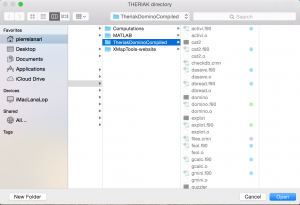Figure 2: Selection of Theriak-Domino directory

• Create a working directory such as UserName/MyDocuments/MATLAB/GRTMOD_Projects/

### 3. Tutorial (GRTMOD demo)

#### 3.1 Stage 1

• Open MATLAB and go to the working directory and the project Demo/ using the “current folder” window
• Open GRTMODin.txt
• Check that the block corresponding to stage 2 is commented: `->> Stage 2`
• Run GRTMOD using the following command in the MATLAB “command window”: ` >> GRTMOD` (Fig. 3)Figure 3: Run GRTMOD in the project directory using the command GRTMOD

• Select the solution to be displayed (Fig. 4). In this example, there is only one solution at T = 752°C and P = 8235 bar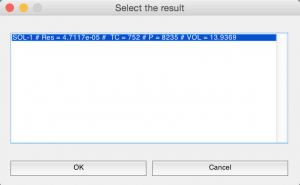Figure 4: Selection of the best solution for stage 1

• Results are printed out into the MATLAB “command window” (see Stage1.txt) and two diagrams are generated (Fig. 5 & Fig. 6)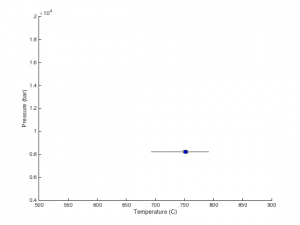Figure 5: P-T diagram for stage 1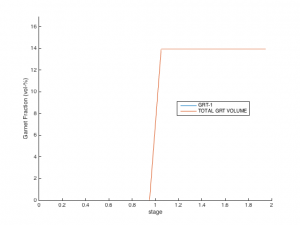Figure 6: Garnet volume fraction diagram

• GRTMOD generates a restart file: RESTART_Stage(1).mat (Fig. 7)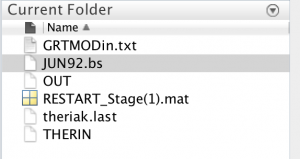Figure 7: Files generated by GRTMOD

#### 3.2 Interpretation of the results for stage 1

The procedure is divided into three phases: optimization1, optimization2 and auto-refinement. During optimization1 successive P-T minimizations are carried out from different starting guesses in order to determine the global minimum within the P-T window. For stages i > 1 The optimization2 refines the garnet fraction variables, as well as P-T. The starting guess for optimization2 is the best P-T couple obtained during optimization1. A go fast mode is available to begin directly optimization2 from user’s favorite P-T initial guess. Finally, the auto-refinement phase checks the local variability of the objective function in order to provide a relative uncertainty on the P-T estimate.

In this example (stage 1) the solution of the RUN 1/4 is selected as the best solution.

#### 3.3 Stage 2

• Open GRTMODin.txt
• It is not required to calculate again the first stage and GRTMOD reads the restart file if the variable COMP of stage 1 is set to 0: `* COMP: 0`
• Activate the stage 2: `>> Stage 2` and set the variable COMP of stage 2 to 1: `* COMP: 1`
• Run GRTMOD using the following command in the MATLAB “command window”: `>> GRTMOD` (Fig. 3)
• Select the second solution SOL-2 at T = 649°C and P = 15335 bar as the best solution (Fig. 8) because a higher garnet volume fraction is predicted (as suggested by the observations).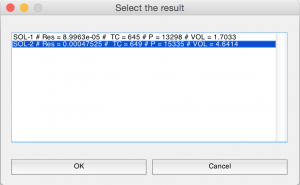Figure 8: Selection of the best solution for stage 2

• Results are printed out into the MATLAB “command window” (see Stage2.txt) and two diagrams are generated (Fig. 9 & Fig. 10)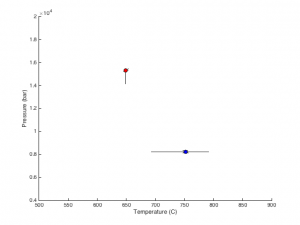Figure 9: P-T diagram after stage 2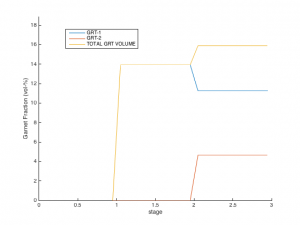Figure 10: Evolution of garnet volume fractions diagram after stage 2

• GRTMOD generates a restart file: RESTART_Stage(2).mat
• A table containing the final results is reported into the MATLAB command window (Fig. 11)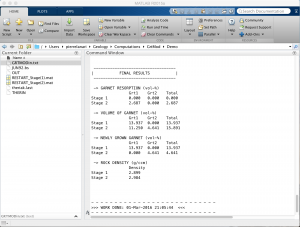Figure 11: Results printed out in the MATLAB command window (after stage 2)

#### 3.4 Interpretation of the results for stage 2

During optimization1, GRTMOD runs 4 minimizations (see input variables for the initial P-T conditions). Two minimizations 1 and 3 fail to find a solution (residue of 1e+19). For run 1, the fraction of garnet predicted stable (0.19872 vol-%) is too small below the lower limit (1%). For run 3, garnet is not predicted stable at the initial P-T conditions of TC = 800°C & P = 8000 bar. By contrast the minimizations 2 and 4 converged to a minimum with residue of 0.00010767 and 0.017066 respectively. The solution of minimization 2 (TC = 645 °C & P = 13296 bar) is selected as the best solution for optimization2.

During optimization2, 3 minimisations are done from the best P-T solution of optimization1 (TC = 645 °C & P = 13296 bar, see above) with variable fraction of Grt1 (X-1), fractionated from the bulk rock composition: 13.9369 vol-% (solution from stage 1 = no resorption); 4 vol-% (strong resorption); 8.9685 vol-% (moderate resorption). RUN 1/3 converges to a solution at TC = 645 °C and P = 13298 bar for a X-1 of 13.9333 vol-% with 1.7033 vol-% of Grt2 stable. RUN 2/3 converges to a solution at TC = 649 °C and P = 15335 bar for a X-1 of 11.25 vol-% with 4.6414 vol-% of Grt2 stable. RUN 3/3 converges to a solution at TC = 649 °C and P = 15335 bar for a X-1 of 8.96 vol-% with 5.3526 vol-% of Grt2 stable. The minimizations 2 and 3 converges to the same solution in P-T, and GRTMOD stored only the solution 2.

Two auto-refinement stages are calculated for the to solutions: solution 1 from RUN 1 and solution 2 from RUN 2.

The second solution is selected as best solution because ~4 vol-% of garnet from that stage is observed in the sample. The growth of Grt2 is modeled with a the resorption of 2.687 vol-% of Grt1 (Fig. 11).

### 4. GRTMOD Command in GRTMODin.txt

#### 4.1 INIT block:

* THDB: Thermodynamic database (JUN92.bs or tc55.txt)

* SAMP: End of theriak default line [* Sample Name] must start with * ! could be used to define set a buffer *,buffer_name

* SYST: SIO2 TIO2 AL2O3 FEO FE2O3 MNO MGO CAO NA2O K2O     ! DO NOT CHANGE …

WARNING: Fe must be defined as FeO in the current version of GRTMOD. FE2O3 should not be used.

* BULK: Define the bulk composition (in oxide wt-%) of the system

* NH2O: h(X) in therin

* NOFI: O(X) in therin (usually “?”) – If this option is not set the value O(?) is applied;

* NCFI: C(X) in therin – If this option is not set the value C(0) is applied;

* NEXO: O(X) in therin – Excess Oxygen as in Thermocalc. NB: If this option is not set the excess O = 0;

* STOL: Tolerance for an acceptable solution (def. 0.05)

* RESC: Residuum criteria to refine errors (def. 0.015)

* SELS: Mode to select the solution:  auto (best),  manual

* SELP: Mode to select the solution to be plotted:  all,  only the selected solution

* TOLX: Optimization parameter: Termination tolerance on x (def. 0.001)

* TOLF: Optimization parameter: Termination tolerance on the function value (def. 0.001)

* DISP: Display optimization info: [off] [iter] [final] [notify]

* TMIN: Set Tmin of the problem (C)

* TMAX: Set Tmax of the problem (C)

* PMIN: Set Pmin of the problem (bar)

* PMAX: Set Pmax of the problem (bar)

* TDI1: T discretization first optimization (C)

* PDI1: P discretization first optimization (bar)

* TDI2: T discretization refinement (C)

* PDI2: P discretization refinement (bar)

#### 4.1 STAGE block:

* COMP: compute  or use a restart .

* OPTI: optimization ( yes;  no;  Go fast mode: run directly opt2 using conditions defined in OPTP)

* REDI; P-T refinement with error estimation ( yes,  no)

* MAPC: map computation between TMIN TMAX and PMIN and PMAX ( using TDI1,  using TDI2)

CVAR: composition variable… [Ref_Stage] is required

* CVAR-GARN-[Ref_Stage]-COMP: Define the garnet oxide wt-% composition (SIO2 TIO2 AL2O3 FEO FE3O3 MNO MGO CAO NA2O K2O, do not change!)

* CVAR-GARN-[Ref_Stage]-WEIG: Define the weights: [wFe wCa wMg wMn]; Basically the number of counts from the X-ray map.

Update (Nov. 2018): If you do not have X-ray maps of garnet, you can use my converter file that generates pseudo X-ray intensities from garnet spot analyses. NOTE: The weighting procedure can significantly affect the results if the weights are not correctly defined.

* CVAR-GARN-[Ref_Stage]-FVOL: Final volume % of this garnet; used by the program the minimum volume fraction of garnet

* CVAR-GARN-[Ref_Stage]-MVOL: Maximal volume % value for this garnet (100% if not defined for stage > 1)

* TRYI: Try to run the optimizations (1 and 2) from the additional initial guess [T P].

Each row starting with the keyboard TRYI defines an additional starting position.

* OPTP: run directly optimization 2 from this starting guess (required if OPTI ==  for this stage)

* MACC: Maximum garnet volume bounds (0 means don’t use it) vector with iStage-1 values – not used for stage 1

* MINC: Minimum garnet volume bounds (0 means don’t use it) vector with iStage-1 values – not used for stage 1